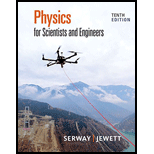# Two parallel wires are separated by 6.00 cm, each carrying 3.00 A of current in the same direction. (a) What is the magnitude of the force per unit length between the wires? (b) Is the force attractive or repulsive?### Physics for Scientists and Enginee...

10th Edition
Raymond A. Serway + 1 other
Publisher: Cengage Learning
ISBN: 9781337553278

#### Solutions

Chapter
Section### Physics for Scientists and Enginee...

10th Edition
Raymond A. Serway + 1 other
Publisher: Cengage Learning
ISBN: 9781337553278
Chapter 29, Problem 13P
Textbook Problem
286 views

## Two parallel wires are separated by 6.00 cm, each carrying 3.00 A of current in the same direction. (a) What is the magnitude of the force per unit length between the wires? (b) Is the force attractive or repulsive?

(a)

To determine
The magnitude of force per unit length between the wires.

### Explanation of Solution

Given info: The distance between the wires is 6.00cm and the magnitude of electric current is 3.00A .

The formula to calculate the magnitude of force per unit length is,

FL=μI1I22πd (1)

Here,

I1 is the electric current in wire 1.

I2 is the electric current in wire 2.

d is the distance between the wires.

μ is the permeability of free space.

The value of I1 is equal to I2 which is 3.00A .

Substitute 4π×107T/mA for μ , 3.00A for I1 , 3.00A for I2 and 6.00cm for d in equation (1) to find the value of FL

(b)

To determine
The force is attractive or repulsive.

### Still sussing out bartleby?

Check out a sample textbook solution.

See a sample solution

#### The Solution to Your Study Problems

Bartleby provides explanations to thousands of textbook problems written by our experts, many with advanced degrees!

Get Started

Find more solutions based on key concepts
What are the features of a healthy high-fat diet?

Understanding Nutrition (MindTap Course List)

The USDA Eating Patterns recommend a small amount of daily oil from which of these sources? a. olives b. nuts c...

Nutrition: Concepts and Controversies - Standalone book (MindTap Course List)

Why do you think the mid-ocean ridges are higher than the surrounding seabed?

Oceanography: An Invitation To Marine Science, Loose-leaf Versin